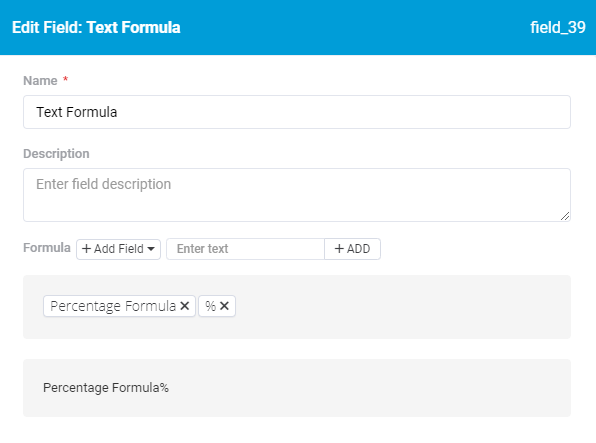# How to display an Equation as %

I have equations that I’d like to display as a percentage in detail component on a page.

Here is one of the equations: {Total Pre-Test Completed}/{Total Submitted}
It displays as .01945467 and I’d like to display is as 19.45%

Does anyone know how to accomplish this?

Thanks!

Hey @mdykstra,

A couple things you could do…

1. Use this as your equation field formula…`({Total Pre-Test Completed}/{Total Submitted})*100` and set the decimal places to 2

1. Use option 1 + a text formula field `{Percentage Formlua}%` You could use this field as a display only field and the actual equation field for calculations since the equation field can be set as a number.1. I just discovered this and started using it. Using an equation field to calculate percentage and simultaneously build an HTML progress bar using the `<progress>` tag.

`CONCAT('<progress',CHAR(32), 'value="',({Total Pre-Test Completed}/{Total Submitted})*100,'"',CHAR(32),'max="100"></progress>')`

1 Like

Thanks @tim.young. I set these up this way, however, the data displayed in the back-end and the front-end is ignoring the 2 decimal places and showing 4.166666666666666 for example.

I have come across this as well. Here is a workaround, use this equation instead:
Round((({Total Pre-Test Completed}/{Total Submitted})*100),2)

Perfect. Thank you TIm and Cam!

1 Like